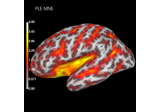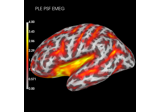# mne.minimum_norm.resolution_metrics#

mne.minimum_norm.resolution_metrics(resmat, src, function='psf', metric='peak_err', threshold=0.5, verbose=None)[source]#

Compute spatial resolution metrics for linear solvers.

Parameters:
resmat`array`, shape (n_orient * n_vertices, n_vertices)

The resolution matrix. If not a square matrix and if the number of rows is a multiple of number of columns (e.g. free or loose orientations), then the Euclidean length per source location is computed (e.g. if inverse operator with free orientations was applied to forward solution with fixed orientations).

srcinstance of `SourceSpaces`

Source space object from forward or inverse operator.

function‘psf’ | ‘ctf’

Whether to compute metrics for columns (point-spread functions, PSFs) or rows (cross-talk functions, CTFs) of the resolution matrix.

metric`str`

The resolution metric to compute. Allowed options are:

Localization-based metrics:

• `'peak_err'` Peak localization error (PLE), Euclidean distance between peak and true source location.

• `'cog_err'` Centre-of-gravity localisation error (CoG), Euclidean distance between CoG and true source location.

Spatial-extent-based metrics:

• `'sd_ext'` Spatial deviation (e.g. ).

• `'maxrad_ext'` Maximum radius to 50% of max amplitude.

Amplitude-based metrics:

• `'peak_amp'` Ratio between absolute maximum amplitudes of peaks per location and maximum peak across locations.

• `'sum_amp'` Ratio between sums of absolute amplitudes.

threshold`float`

Amplitude fraction threshold for spatial extent metric ‘maxrad_ext’. Defaults to 0.5.

verbose

Control verbosity of the logging output. If `None`, use the default verbosity level. See the logging documentation and `mne.verbose()` for details. Should only be passed as a keyword argument.

Returns:
resolution_metricinstance of `SourceEstimate`

The resolution metric.

Notes

For details, see .

New in version 0.20.

References

## Examples using `mne.minimum_norm.resolution_metrics`#Compute spatial resolution metrics in source space

Compute spatial resolution metrics in source spaceCompute spatial resolution metrics to compare MEG with EEG+MEG

Compute spatial resolution metrics to compare MEG with EEG+MEG